# KSEEB Solutions for Class 8 Maths Chapter 5 Squares, Square Roots, Cubes, Cube Roots Ex 5.4

In this chapter, we provide KSEEB SSLC Class 8 Maths Chapter 5 Squares, Square Roots, Cubes, Cube Roots Ex 5.4 for English medium students, Which will very helpful for every student in their exams. Students can download the latest KSEEB SSLC Class 8 Maths Chapter 5 Squares, Square Roots, Cubes, Cube Roots Ex 5.4 pdf, free KSEEB SSLC Class 8 Maths Chapter 5 Squares, Square Roots, Cubes, Cube Roots Ex 5.4 pdf download. Now you will get step by step solution to each question.

## Karnataka Board Class 8 Maths Chapter 5 Squares, Square Roots, Cubes, Cube Roots Ex 5.4

1. Find the square root of the following numbers by factorization.

Question (i)
196
196 = 2 × 2 × 7 × 7
= 2 × 7 × 2 × 7
196 = 14 × 14
√196 = 14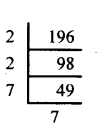Question (ii)
256
256 = 2 × 2 × 2 × 2 × 2 × 2 × 2 × 2
256 = 16 × 16
√256 = 16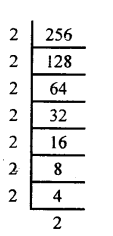Question (iii)
10404
10404 = 2 × 2 × 3 × 3 × 17 × 17
= 2 × 3 × 17 × 2 × 17
10404 = 102 × 102
√10404 = 102Question (iv)
1156
1156 = 2 × 2 × 17 × 17
= 2 × 17 × 2 × 17
1156 = 34 × 34
√1156 = 34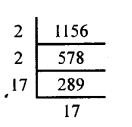Question (v)
13225
13225 = 5 × 5 × 23 × 23
= 5 × 23 × 5 × 23
13225 = 115 × 115 7
√13225 = 115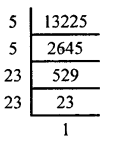2. Simplify

Question (i)
√100 + √36
√100 + √36 = 10 + 6 = 16

Question (ii)
√1360 + 9
√1360 + 9 = √1369
√1369 = 37 × 37
√1369 = 37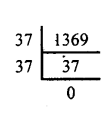Question (iii)
√2704+√144+√289
2704 = 2 × 2 × 2 × 2 × 13 × 13
= 2 × 2 × 13 × 2 × 2 × 13
2704 = 52 × 52
√2704 =52
√144 = 12 and √289 = 17
∴ √2704 + √144 + √289 = 52 + 12 + 17 = 81

Question (iv)
√225 – √25
15 – 5=10

Question (v)
√1764 – √1444
1764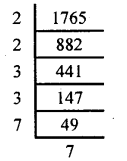1764 = 2 × 2 × 3 × 3 × 7 × 7
=2 × 3 × 7 × 2 × 3 × 7
1764 = 42 × 42
√l764 = 42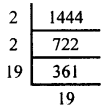1444 = 2 × 2 × 19 × 19
= 2 × 19 × 2 × 19
1444 = 38 × 38
√1444 = 38
∴√l764 – √1444 = 42 – 38 = 4

Question (vi)
√169 × √361
√169 × √361 = 13 × 19 = 247

Question 3.
A square yard has an area 1764 m2. From a corner of this yard, another square part of area 784 m2 is taken out for public utility. The remaining portion is divided into equal square parts. What is the perimeter of each of these equal parts?
The area of the remaining portion= 1764 – 784 = 980 m2
It is divided into 5, equal square parts
∴ Each square part = 9805 = 196 m2
Area of each square part = 196 m2
(side)2 = 196
side = √196
side = 14.
The length of each side of the square = 14m
Perimeter = 4 side = 4 × 14 = 56m

4. Find the smallest positive integer with which one has to multiply each of the following numbers to get a perfect square.

Question (i)
847
847 = 7 × 11 × 11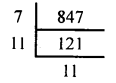Here we observe that 7 appears only once.
∴The required number of multiplied is 7.

Question (ii)
450
450 = 2 × 5 × 5 × 3 × 3
Here we observe that 2 appears only once
∴ The required number is 2.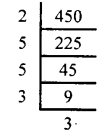Question (iii)
1445
Answer: 1445 = 5 × 17 × 17
Here we observe that 5 appears only once
∴ The required number of multiplied is 5.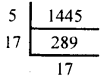Question (iv)
1352
1352 = 2 × 2 × 2 × 13 × 13
= 2 × 13 × 2 × 13 × 2
= 26 × 26 × 2
Here we observe that 2 appears only once in the product.
∴ The required number multiplier is 2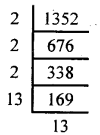5. Find the largest perfect square factor of each of the following numbers.

Question (i)
48
48 = 2 × 2 × 2 × 2 × 3
48= 16 × 3
∴ Largest perfect square factor is 16.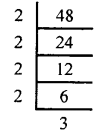Question (ii)
11280
11280 = 2 × 2 × 2 × 2 × 3 × 5 × 47
= 16 × 3 × 5 × 47
∴ Largest perfect square factor is 16.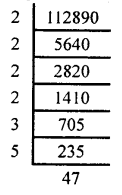Question (iii)
729
729 = 3 × 3 × 3 × 3 × 3 × 3
= 27 × 27
= 729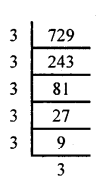∴ Largest perfect square factor is 729.

Question (iv)
1352
Answer: 1352 = 2 × 2 × 2 × 13 × 13
= 2 × 13 × 2 × 3 × 2
= 26 × 26 × 2
= 676 × 2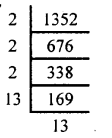∴ The largest perfect square factor is 676

All Chapter KSEEB Solutions For Class 8 maths

—————————————————————————–

All Subject KSEEB Solutions For Class 9

*************************************************

I think you got complete solutions for this chapter. If You have any queries regarding this chapter, please comment on the below section our subject teacher will answer you. We tried our best to give complete solutions so you got good marks in your exam.

If these solutions have helped you, you can also share kseebsolutionsfor.com to your friends.

Best of Luck!!